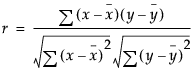Publication date: 08/13/2020

## Pearson Product-Moment Correlation

The Pearson product-moment correlation coefficient measures the strength of the linear relationship between two variables. For response variables X and Y, it is denoted as r and computed as follows:If there is an exact linear relationship between two variables, the correlation is 1 or –1, depending on whether the variables are positively or negatively related. If there is no linear relationship, the correlation tends toward zero.